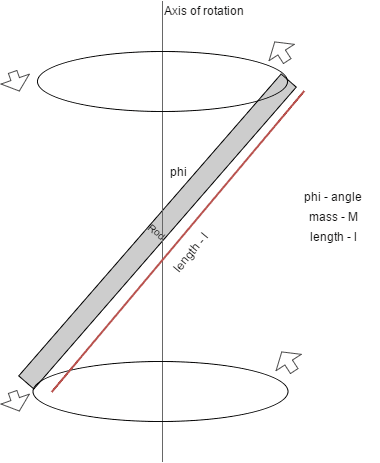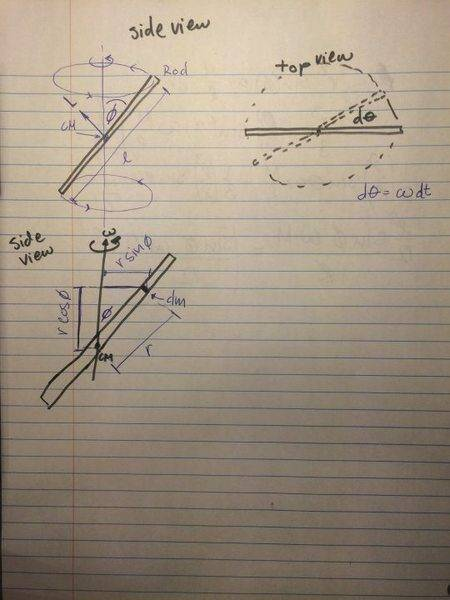# Angular Momentum - rotating rod makes an angle phi with axis

• ben3
L = r × vdm... is the magnitude of the angular momentum. dL = r × vdm... is the direction of the angular momentum.f

## Homework Statement

A thin rod of length l and mass M rotates about a vertical axis through its center with angular velocity ω. The rod makes an angle φ with the rotation axis. Determine the magnitude and direction of L (angular momentum).

So we're given: mass - M, length - l, angular velocity - ω, angle - φ

2. Homework Equations

I know that I have to use L = r × p = r × vm
In this case L = I⋅ω is not valid because L and ω vectors are not perpendicular

## The Attempt at a Solution

So it tried this...
I used the L = r × vm
but because my object is a rod with uniform mass I take the derivative of L = r × vm to get dL = r × vdm

because it's a derivative I have an infinitely small mass dm, so I said that dm=(M/l)dr

r is now the radius of a point mass on the rod from the center of mass

v is the velocity of that point mass, and since we know ω we can use v = ωr
and so I get that v = (r sinφ)ω [rsinφ is the perpendicular distance to dm from the axis]

1. I start here
dL = r × vdm

2. I now integrate both sides to get L
∫ dL = ∫ r(rsinf)wdm =

3. I integrate from -l/2 to l/2 because that is the length of the rod

L = ∫1/2-1/2 (r sinφ) w(M/l)dr

4. I now plug in the two values
L = (r3/3)(sinφ)(ωM/l) |1/2-1/2

so after plugging everything in I get: L = (sinφ ω M l2)/12

MY QUESTION: IS THIS CORRECT? I did a lot of guessing because I could not find anything similar to this in my physics book or online.

1. Is my thinking correct?
2. Am I allowed to integrate ∫
r(rsinf)wdm because r × vm is a cross product.

(diagram are included)Thanks

You are asked to find the magnitude and direction of the angular momentum. By choosing a specific axis as the axis of rotation you are dictating the direction.
Ask yourself this: would the rod maintain this motion in free fall? If not, there must be forces, in particular a torque, maintaining it. If there is a torque, is the L vector constant?

Start with the rod's centre as origin. Pick a small element of the rod at some distance from it. If r is the vector of that position, what is the angle between that vector and the velocity of the element? In what direction is the cross product?

You are asked to find the magnitude and direction of the angular momentum. By choosing a specific axis as the axis of rotation you are dictating the direction.
Ask yourself this: would the rod maintain this motion in free fall? If not, there must be forces, in particular a torque, maintaining it. If there is a torque, is the L vector constant?
If there is torque than L is not constant. But then you have torque on both sides of the rod so net torque is zero?

If r is the vector of that position, what is the angle between that vector and the velocity of the element?
The r vector and velocity vector should be perpendicular to each other, so at the angle of 90 degrees?

In what direction is the cross product?
Well cross product is always in the plane perpendicular to the two vectors?

##dL = \vec r \times \vec v(r)\; dm##
Try doing the cross product explicitly ... ##\vec v(r)## is constantly changing direction, but the relative orientations of ##\vec v## and ##\vec r## don't change.
For simplicity pick an orientation so that the rod lies in the x-z plane. Express the vectors in x-y-z components. Do the cross product and see what happens.

If there is torque than L is not constant. But then you have torque on both sides of the rod so net torque is zero?
It is given that the rod rotates about the z axis. It is forced to rotate about the axis by the bearing, or some other kind of external torque.
The angular momentum of a point mass is the vector product L=m[rxv]. v is horizontal, but r has both horizontal (perpendicular to v) and vertical components.
The r vector and velocity vector should be perpendicular to each other, so at the angle of 90 degrees?
Yes, this is true.

Well cross product is always in the plane perpendicular to the two vectors?
The cross product is axb is perpendicular to both a and b .

Okay so this is what I imagine the problem looks like. Velocity vector V is pointing into the paper and r has an x and y component and then Torque T is perpendicular to both V and r

This is correct right?
You mean that the angular momentum is perpendicular to both r and v.
You can get the components of the angular momentum, as Simon suggested. One component changes with time as the rod rotates, the other is constant.
Also remember that the magnitude of the cross product |axb| = a*b sin(θ) where θ is the angle between a and b. You know this angle , so you got the magnitude of the angular momentum already in your first post.

•ben3
Ok I think I finally understand this.

I first wrote r and v as:
r = [r*sin(φ)*cos(ωt)]i + [r*sin(φ)*sin(ωt)]j + [r*cosφ]k
=r
[[sin(φ)*cos(ωt)]i + [sin(φ)*sin(ωt)]j + [cosφ]k]

so r*sin(φ) is the horizontal radius from the point mass to the axis of rotation and
cos(ωt) accounts for the mass rotating, so it will change with time (I got that from saying that θ = ωt)
r*cosφ is just the height from the center of mass

then velocity is the derivative of position/radius v = dr/dt so i just derive r to get v, i get:
v = [-rω*sinφ*sinωt]i + [rω*sinφ*cosωt]j
=
rω[[-sinφ*sinωt]i + [sinφ*cosωt]j ]

so since now i have both r and v i can plug them in dL = r × vdm like Simon suggested and I also plugged in dm=(M/l)dr
and so after the cross product and after integration from -l/2 to l/2 I got L:
L = (1/12)(Mωsinφl2)[(-cos(ω) cos(ωt))i - (cos φsin (ωt))j + (sin φ)h]

Now I'm just need to get rid of i j and k how could i do that?

L = (1/12)(Mωsinφl2)[(-cos(ω) cos(ωt))i - (cos φsin (ωt))j + (sin φ)h]

Now I'm just need to get rid of i j and k how could i do that?
The cos(ω) should be cos(φ).
How do you find the magnitude of a vector from its Cartesian coordinates?

•ben3
Ohh i see! I just use Pythagorean theorem, so I square i, j, and k and take the square root and so the second part becomes 1 because everything cancels out!

so final result is L = (1/12)(Mωsinφl2)

Thanks everyone for helping!

Ohh i see! I just use Pythagorean theorem, so I square i, j, and k and take the square root and so the second part becomes 1 because everything cancels out!

so final result is L = (1/12)(Mωsinφl2)

Thanks everyone for helping!
This is the magnitude (if l2 means l2). But what is the direction of L?